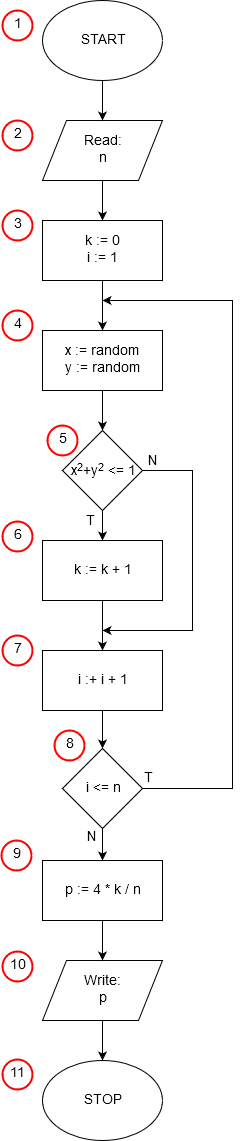# Monte Carlo Calculation of pi in Pascal

<<algorithmprogram Number_pi; var i, n, k: integer; x, y, p: double; begin write('n = '); readln(n); randomize; k:=0; for i:=1 to n do begin x:=random; y:=random; if (x*x + y*y)<=1 then k:= k+1; end; p:=4*k/n; writeln('pi = ', p:10:8); readln; end.

The first line of the program can be treated as an ornament but required by pascal syntax. Every program in the pascal must start with the keyword program preceding the program name, it does not contribute anything to the implementation of the algorithm. Third and fourth lines are auxiliary. We know that our algorithm uses three variables that store integers and three variables that store real numbers. In the pascal, all variables must be declared before the code block starts. Declaring a variable we have to specify what type of data will be stored in it. Variable declaration starts with var keyword.

The sixth line containing the keyword begin corresponds to the startup block (1) of our algorithm.

Lines 7 and 8 perform an input/output operation (2) in which input data is taken - the number of random points n.

In line 9 we call the function randomize from the standard library. This is a function that initializes the built-in pseudorandom number generator by a random value generated from the current time when the contents of the system clock are executing. If we did not call this function, the program would generate the same sequence of pseudorandom numbers each time we started, and as a result we would always get the same result for a given number of random points. In every Pascal program in which we use the pseudorandom number generator, we should call the randomize function before the first randomization.

In line 10 we reset k - counter of points lying in circle with radius 1 (3).

Line 11 starts the for loop instruction. The instructions between the begin and end keywords will be executed n times (the control variable i of the loop will change from 1 to n, each time increasing its value by 1). Line 11 implements part of point (3) of the algorithm, initializing the variable i of value 1 and execute points (7) and (8) of the algorithm, incrementing after each instruction execution loop control variable i by 1 and checking whether the value of the number has not yet exceeded the upper limit of the loop (i <= n). Inside the for loop always has exactly one instruction. As we need here, as in our example, more instructions, we need to write them between the begin and end keywords as a compound statement.

The instructions in the loop are contained in lines 12-14. For each random point we first draw lots its coordinates x and y - point (4) of the algorithm. We use a random function library for the randomization, which results in a double random (pseudorandom) number from 0 to 1. Then, in line 14, we check that the point with the drawn x, y lies in the circle with radius 1 (5). If this is the case, then the content of the variable k is 1 (6).

At the end of the loop, the variable n contains the total number of points drawn (the content of the variable n has not changed) and the variable k contains information about the number of points drawn in the circle with radius 1.

In line 16, we assign an approximate value of π (9) to the variable p, and then print the result obtained (input-output block (10)) in line 17.

The readln instruction in line 18 has no relation to the implementation of the algorithm. It is used to stop the program until you press the enter key, preventing immediate closure of the program window.

Instruction end. in line 19 ends the program (end of the algorithm block (11)).